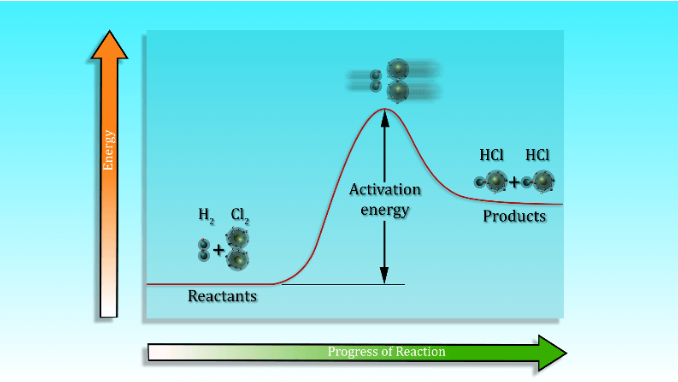Activation Energy

What is Activation Energy?

Activation Energy refers to the energy that must be provided to potential reactants in a chemical or a nuclear system in order to spark a chemical reaction or a nuclear reaction. Activation energy is generally denoted by ‘Ea‘ and is measured in terms of energy per mole of reactant (such as kilojoules/mole or kilocalories/mole). It can also be measured using joules per mole.

Activation energy can be visualized as the magnitude of the energy barrier (or the potential barrier) which separates the minima of potential energy surfaces involving the initial and the final thermodynamic state of the system. This concept was first introduced by Swedish Chemist Svante August Arrhenius in the year 1889. A graph illustrating the activation energy of a reaction is provided below.It can be noted that the activation energies of different reactions vary. This energy requirement can be affected by the temperature of the system and also by the presence of a catalyst.

Temperature Dependence of the Rate of Reaction

From the Arrhenius equation, the following equation can be considered in order to calculate the activation energy of a system:

$k = Ae^{\frac{-E_{a}}{RT}}$

Wherein: k refers to the rate constant, T refers to the absolute temperature, R is the universal gas constant, and A refers to the frequency of correctly oriented collisions. Ea is the activation energy as discussed earlier.

Negative Activation Energies

• A negative value of activation energy is found in the Arrhenius equations of reactions wherein the rate of reaction slows down due to an increase in temperature.
• In general, reactions which show a negative value for the activation energy are barrierless reactions which involve the capture of molecules in a given potential well.
• An increase in the temperature for these reactions results in a reduction in the probability of the colliding molecules to capture each other.

Effect of Catalysts on Activation Energy

Catalysts are basically substances that increase the rate of the reaction they are subjected to without being consumed by the reaction while accomplishing this. These substances work to modify the transition states of reactions in order to lower the activation energies of the aforementioned reactions.

An enzyme is a specific type of catalyst which is made up of only proteins and small molecule cofactors.

It is important to note that while the action of catalysts reduces the overall activation energy of the reaction, it has no effect on the energies of the reactants or products of the reaction in question. Only the Ea of the reactions is altered by these catalytic actions.

Activation Energies of Endothermic and Exothermic Reactions

Reactions can be broadly classified as endothermic or exothermic reactions based on the role of utilization of thermal energy in these reactions.

Endothermic Reactions

• The reactions that require absorption of thermal energy by the reactants to form the necessary products are termed endothermic reactions.
• These types of reactions typically have higher activation energies and also require a constant input of energy even after the Ea is provided. A graph detailing the energy requirements of these reactions is illustrated below.
• An example of an endothermic reaction is the melting of ice to form water.

Exothermic Reactions

The reactions that release thermal energy during the formation of products are referred to as exothermic reactions. These types of reactions generally have low activation energies and they do not require a constant input of energy such as in the case of endothermic reactions. A graph of the energy requirements of these reactions can be illustrated as follows.

An example of this type of reaction is the combustion of fuel in a bunsen burner. The sparks which need to be provided to start the flame provide the required activation energy to the reacting fuel and the reaction sustains itself after this initial spark is provided.

To learn more about activation energy and other important concepts related to chemical kinetics, register with BYJU’S and download the mobile application on your smartphone.